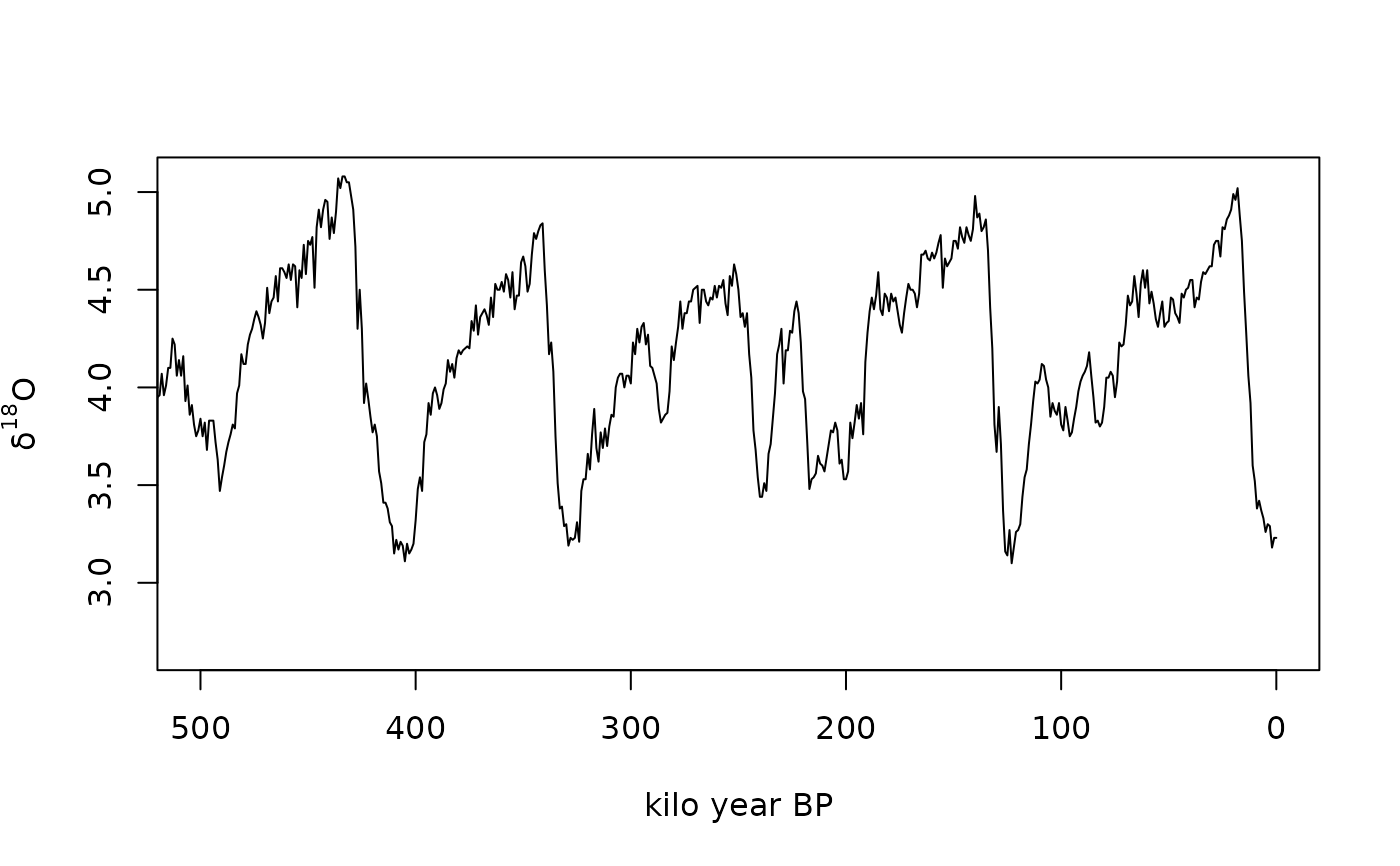A global Pliocene-Pleistocene benthic $$\delta^{18}O$$ stack.

lisiecki2005

Format

A data frame with 3 variables:

age

Calendar (calibrated) age (kilo year).

delta

Benthic $$\delta^{18}O$$ (per mil).

error

Standard error (per mil).

Details

The LR04 stack spans 5.3 Myr and is an average of 57 globally distributed benthic $$\delta^{18}O$$ records (which measure global ice volume and deep ocean temperature) collected from the scientific literature.

Lisiecki, L. E. and Raymo, M. E. (2005). A Pliocene-Pleistocene stack of 57 globally distributed benthic d18O records. Paleoceanography, 20, PA1003. doi: 10.1029/2004PA001071

Examples

plot(
x = lisiecki2005$age, y = lisiecki2005$delta,
type = "l",
xlim = c(500, 0),
xlab = "kilo year BP",
ylab = expression(delta^{18}*"O")
)